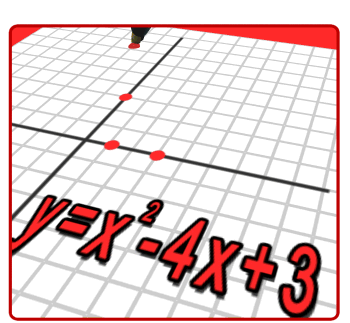• Mrs Vitagliano's 7th/8th Grade Math Classes

"Once we accept our limits, we go beyond them."  …………Albert Einstein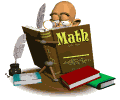Students in grade 7/8 will extend their understanding of ratios and develop understanding of proportionality to solve single- and multi-step problems pertaining to the real-world.  This understanding of ratios and proportionality will aid the students in solving a wide variety of percent problems, including those involving discounts, interest, taxes, tips, and percent increase or decrease.  Students will also graph proportional relationships and understand the unit rate as a measure of the steepness of the related line, called slope.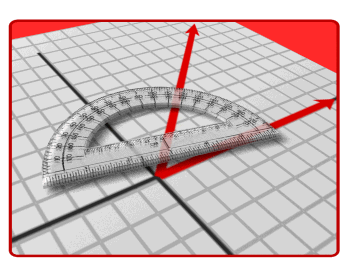Students will develop a unified understanding of number, recognizing fractions, decimals (finite or repeating), and percents as different representations of rational numbers.  Students will extend operations to all rational numbers while maintaining all properties.  They will use the arithmetic of rational numbers as they formulate expressions and equations in one variable and use these equations to solve problems.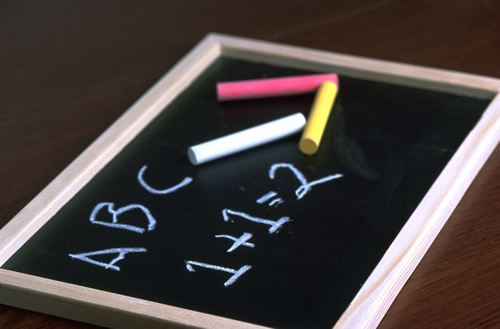Students build on their skills in Geometry, solving problems involving the area and circumference of a circle and surface area of three-dimensional objects.  Past knowledge of congruence and similarity will help the students reason about relationships among two-dimensional figures using scale drawings and informal geometric constructions, and they will gain familiarity with the relationships between angles formed by intersecting lines.  Students will work with three-dimensional figures, relating them to two-dimensional figures by examining cross-sections.  They solve real-world problems involving area, surface area, and volume of two and three dimensional objects.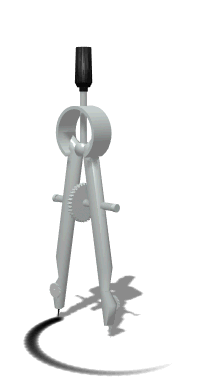Lastly, students will draw informal comparative inferences to compare data distributions and address questions about differences between populations.   They begin informal work with random sampling to generate data sets and learn about the importance of representative samples for drawing inferences.